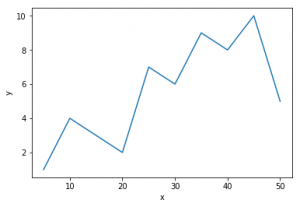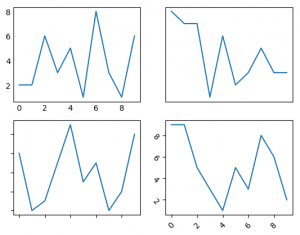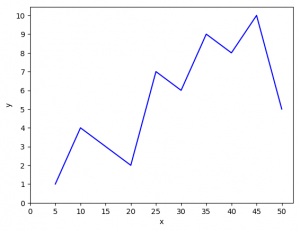GeeksforGeeks App
Open AppBrowser
Continue

# Python | Matplotlib.pyplot ticks

Matplotlib is an amazing visualization library in Python for 2D plots of arrays. Matplotlib is a multi-platform data visualization library built on NumPy arrays and designed to work with the broader SciPy stack. It was introduced by John Hunter in the year 2003.
One of the greatest benefits of visualization is that it allows us visual access to huge amounts of data in easily digestible visuals. Matplotlib consists of several plots like line, bar, scatter, histogram etc.
Ticks are the values used to show specific points on the coordinate axis. It can be a number or a string. Whenever we plot a graph, the axes adjust and take the default ticks. Matplotlib’s default ticks are generally sufficient in common situations but are in no way optimal for every plot. Here, we will see how to customize these ticks as per our need.
Parameters

Example #1: Default plot

## Python3

 `# importing required modules``import` `matplotlib.pyplot as plt` `# values of x and y axes``x ``=` `[``5``, ``10``, ``15``, ``20``, ``25``, ``30``, ``35``, ``40``, ``45``, ``50``]``y ``=` `[``1``, ``4``, ``3``, ``2``, ``7``, ``6``, ``9``, ``8``, ``10``, ``5``]` `plt.plot(x, y)``plt.xlabel(``'x'``)``plt.ylabel(``'y'``)` `plt.show()`

Output :Example #2: Playing with the ticks
Suppose we don’t want to display the values of ticks or want our ticks to be tilted or want any other customization. We can do it this way.

## Python

 `# importing libraries``import` `random``import` `matplotlib.pyplot as plt` `fig ``=` `plt.figure()` `# function to get random values for graph``def` `get_graphs():``    ``xs ``=``[]``    ``ys ``=``[]``    ``for` `i ``in` `range``(``10``):``        ``xs.append(i)``        ``ys.append(random.randrange(``10``))``    ``return` `xs, ys` `# defining subplots``ax1 ``=` `fig.add_subplot(``221``)``ax2 ``=` `fig.add_subplot(``222``)``ax3 ``=` `fig.add_subplot(``223``)``ax4 ``=` `fig.add_subplot(``224``)` `# hiding the marker on axis``x, y ``=` `get_graphs()``ax1.plot(x, y)``ax1.tick_params(axis ``=``'both'``, which ``=``'both'``, length ``=` `0``)` `# One can also change marker length``# by setting (length = any float value)` `# hiding the ticks and markers``x, y ``=` `get_graphs()``ax2.plot(x, y)``ax2.axes.get_xaxis().set_visible(``False``)``ax2.axes.get_yaxis().set_visible(``False``)` `# hiding the values and displaying the marker``x, y ``=` `get_graphs()``ax3.plot(x, y)``ax3.yaxis.set_major_formatter(plt.NullFormatter())``ax3.xaxis.set_major_formatter(plt.NullFormatter())` `# tilting the ticks (usually needed when``# the ticks are densely populated)``x, y ``=` `get_graphs()``ax4.plot(x, y)``ax4.tick_params(axis ``=``'x'``, rotation ``=` `45``)``ax4.tick_params(axis ``=``'y'``, rotation ``=``-``45``)``    ` `plt.show()`

Output:Example #3: Changing the values of ticks.
In the first example, the x-axis and y-axis were divided by the value of 10 and 2 respectively. Let’s make it 5 and 1.

## Python3

 `# importing libraries``import` `matplotlib.pyplot as plt``import` `numpy as np` `# values of x and y axes``x ``=` `[``5``, ``10``, ``15``, ``20``, ``25``, ``30``, ``35``, ``40``, ``45``, ``50``]``y ``=` `[``1``, ``4``, ``3``, ``2``, ``7``, ``6``, ``9``, ``8``, ``10``, ``5``]` `plt.plot(x, y, ``'b'``)``plt.xlabel(``'x'``)``plt.ylabel(``'y'``)` `# 0 is the initial value, 51 is the final value``# (last value is not taken) and 5 is the difference``# of values between two consecutive ticks``plt.xticks(np.arange(``0``, ``51``, ``5``))``plt.yticks(np.arange(``0``, ``11``, ``1``))``plt.show()`

Output:The main difference from the 1st example is :
plt.xticks(np.arange(0, 51, 5))
plt.yticks(np.arange(0, 11, 1))
Changing the values in np.arange will change the range of ticks.

Reference: Matplotlib ticks.

My Personal Notes arrow_drop_up